# Reasoning Quiz for SSC CGL Exam 2020: 12th February 2020 For Mathematical Operation

Q1. If “A” denotes “subtracted from”, “B” denotes “added to”, “C” denotes “divided by”, “D” denotes “multiplied by”, then which of the following statement is correct?
(a) 3 A 12 B 16 D 17 C 1 = 163
(b) 5 C 7 A 9 D 8 B 2 = 294
(c) 13 C 13 A 13 B 13 D 13 = 157
(d) 18 C 16 D 49 A 27 B 9 = 200

Q2. In the following question, correct the equation by interchanging two signs.
7 × 6 + 5 – 12 ÷ 3 = 41
(a) + and ÷
(b) × and ÷
(c) + and –
(d) × and –

Q3. If 15 (196) 29 and 16 (100) 6, then what is the value of ‘A’ in 31 (A) 48?
(a) 361
(b) 256
(c) 324
(d) 289

Q4. Change the sign to find the equation 28–7+2 × 2=0
(a) Change + into ×
(b) Change × into +
(c) Change – into +
(d) Change + into –Q5. After interchanging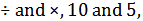which one of the following becomes a correct equation?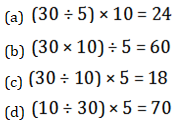Q6. In the following question, correct the equation by interchanging two signs.
18 ÷ 3 + 9 – 6 × 3 = 15
(a) – and ×
(b) + and –
(c) ÷ and –
(d) + and ÷

SSC CGL | SSC CHSL 2020 | Non Verbal (Part 2) | Reasoning Tricks

Q7. If “P” denotes “added to”, “Q” denotes “subtracted from”, “R” denotes “divided by” and “S” denotes “multiplied by”, then which of the following equation is true?
(a) 18 S 12 R 4 P 5 Q 6 = 65
(b) 4 P 57 R 3 Q 7 S 3 = – 2
(c) 6 P 7 Q 12 R 6 S 3 = 7
(d) 29 Q 28 R 2 P 3 S 2 = 10

Q8. If 436 = 3, 783 = 8 and 654 = 5, then 896 = ?
(a) 5
(b) 8
(c)13
(d) 6

Q9. If 32 + 21 = 2809 and 27 + 15 = 1764, then 31 + 13 =?
(a) 1444
(b) 2116
(c) 1936
(d) 2304

Direction (10):- In the following question, by using which mathematical operators will the expression become correct?

Q10. 69 ?3 ?2 ?9 ? 3
(a) ÷, –, > and ×
(b) ÷, +, < and ×
(c) ÷, –, = and ×
(d) ×, +, < and ×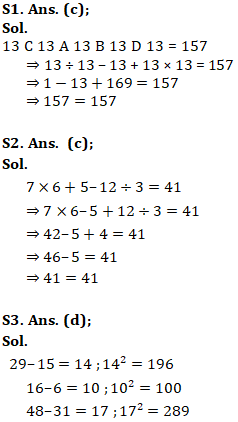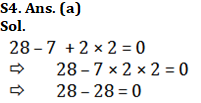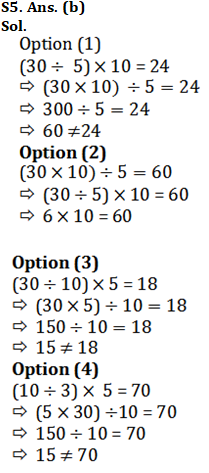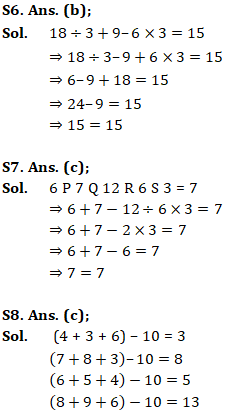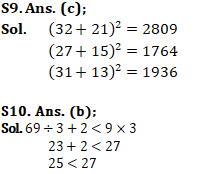You may also like to read:

×

## Download success!

Thanks for downloading the guide. For similar guides, free study material, quizzes, videos and job alerts you can download the Adda247 app from play store.

Thank You, Your details have been submitted we will get back to you.

## Leave a commentJoin India's largest learning destination

What You Will get ?

•Job Alerts
•Daily Quizes
•Subject-Wise Quizes
•Current Affairs
•Previous year question papers
•Doubt Solving session

## Login

OR

Forgot Password?Join India's largest learning destination

What You Will get ?

•Job Alerts
•Daily Quizes
•Subject-Wise Quizes
•Current Affairs
•Previous year question papers
•Doubt Solving session

## Sign Up

ORJoin India's largest learning destination

What You Will get ?

•Job Alerts
•Daily Quizes
•Subject-Wise Quizes
•Current Affairs
•Previous year question papers
•Doubt Solving session

## Forgot Password

Enter the email address associated with your account, and we'll email you an OTP to verify it's you.Join India's largest learning destination

What You Will get ?

•Job Alerts
•Daily Quizes
•Subject-Wise Quizes
•Current Affairs
•Previous year question papers
•Doubt Solving session

## Enter OTP

Please enter the OTP sent to
/6

Did not recive OTP?

Resend in 60sJoin India's largest learning destination

What You Will get ?

•Job Alerts
•Daily Quizes
•Subject-Wise Quizes
•Current Affairs
•Previous year question papers
•Doubt Solving session

## Change PasswordJoin India's largest learning destination

What You Will get ?

•Job Alerts
•Daily Quizes
•Subject-Wise Quizes
•Current Affairs
•Previous year question papers
•Doubt Solving session

## Almost there

Please enter your phone no. to proceed
+91Join India's largest learning destination

What You Will get ?

•Job Alerts
•Daily Quizes
•Subject-Wise Quizes
•Current Affairs
•Previous year question papers
•Doubt Solving session

## Enter OTP

Please enter the OTP sent to Edit Number

Did not recive OTP?

Resend 60

## By skipping this step you will not recieve any free content avalaible on adda247, also you will miss onto notification and job alerts

Are you sure you want to skip this step?

## By skipping this step you will not recieve any free content avalaible on adda247, also you will miss onto notification and job alerts

Are you sure you want to skip this step?